# Vision. Mission. Strategy. Tactics? Values?

I have a love-hate relationship with visions and missions. The ones that companies and organizations spend a lot of time and ressources on developing. They often have a rather formulaic form:

We exist to restore intellectual capital whilst continuing to collaboratively coordinate information.

This is actually a mission statement from a mission statement generator.

I do love the idea of missions, visions and strategies. It speaks to the engineer in me. We should define the goal we want to achieve, and then break that goal down into individual work-packages. When we have completed all of them, we have achieved our goal. The logical framework approach is a good example.

I also like values. I need consistency. Or, I don’t need it, but it is important to me. I like people and organizations to actually be consistent in their actions. Walk the talk! If you want a work environment that does not discriminate – do not discriminate. Anyone. In any way, I do not really mind that you discriminate based on gender. Go ahead. Just be honest about it. In reality, I will of course hate you if you discriminate based on gender. But the hate will take a more deep and incandecent nature if you discriminate based on gender, while claiming that you are all about equality.

So – I love strategies. I love visions. I love missions. I love values.

On the other hand. I hate them. Most of them are exactly like the example above. An example that is taken from the mission statement generator. Noone is able to explain the difference between mission and vision, and the values all falls for the negation test. And tend to end up being rather tautological. Often they are self contradictory. You can have loyalty in all situations. Or you can have honesty in all situations. But you can’t have both. Sometimes being loyal means holding back on honesty a bit. How do you prioritize your values? I have never seen a description of a hierarchy.

Usually it doesn’t matter. Most values in organizations tend to be nothing more than hot air. And as hot air, easy to dismiss when it is opportune. Try it yourself. Tell your boss that her decision is wrong, because it is in conflict the the defined values of the organization.

So – I love values. But not when they are meaningless.

And if they are – don’t bother defining them. They are just going to be a waste of time.

# The Mann-Whitney U test,

also known as the Mann-Whitney-Wilcoxon test, is a non-parametric test of a null hypothesis, that it is equally likely, that a randomly selected value from one sample, will be less than, or greater, than a randomly selected value from a second sample.

Intuitively I think I might be able to see how this translates to “the two samples are from populations that are actually different”. I have a harder time to describe that intuition.

The test is nearly as efficient as a t-test. But the advantadge is, that it does not require that we can assume that the two samples are normal distributed.

It can also be used to test if two independent samples, were selected from populations that have the same distribution.

The idea is, we have to samples. If we pick a random value from sample A, and a random value from sample B, there is a likelihood, a chance, that the value from sample A is larger than the value from sample B. Is that likelihood the same as the likelihood that the value from sample A is smaller than the value from sample B.

# How to do that in R?

Let us look at some data. mtcars is a dataset with some facts about various 1974 US cars.
One of the numbers we have i mpg, miles pr gallon, or fuel-efficiency. Another is about the transmission. Is there a manual or an automatic gearbox. In the column am a “1” indicates an automatic gearbox. A “0” a manual gearbox.

We can now extract two sets of data on the fuel efficiency, based on the type of transmission:

aut <- mtcars[which(mtcars$am==1),]$mpg
man <- mtcars[which(mtcars$am==0),]$mpg


In “aut” we have the fuel efficiency of cars with an automatic gearbox, and in “man” the same, but for the, in Europe, more familiar, manual gearbox.

How to do the Wilcoxon test?

wilcox.test(aut,man)

## Warning in wilcox.test.default(aut, man): cannot compute exact p-value with
## ties

##
##  Wilcoxon rank sum test with continuity correction
##
## data:  aut and man
## W = 205, p-value = 0.001871
## alternative hypothesis: true location shift is not equal to 0


The p-value is 0.001871. If we take a 0.05 significance level, we can conclude that the two sets of values are from non-identical populations. Had it been larger (quite a bit larger), we would not have been able to reject the null-hypothesis, that the two samples comes from identical populations.

There is a different way to do the test, where we dont split the dataset in two:

wilcox.test(mpg ~ am, data=mtcars)

## Warning in wilcox.test.default(x = c(21.4, 18.7, 18.1, 14.3, 24.4, 22.8, :
## cannot compute exact p-value with ties

##
##  Wilcoxon rank sum test with continuity correction
##
## data:  mpg by am
## W = 42, p-value = 0.001871
## alternative hypothesis: true location shift is not equal to 0


We tell the function that we want to test mpg (fuel efficiency), as a function of am (the type of transmission), and that the dataset we are working on is mtcars. The function then splits the dataset in two based on the value in am. Had there been more than two, things would probably stop working.

The result is the same though.

# The warning

We get a warning. That is because the algorithm has a problem when there are identical values in the two datasets. There is a way around. We can add a “jitter”, to the two datasets. jitter() adds small random values to the values in the dataset. Often that solves the problem:

wilcox.test(jitter(aut),jitter(man))

##
##  Wilcoxon rank sum test
##
## data:  jitter(aut) and jitter(man)
## W = 205, p-value = 0.001214
## alternative hypothesis: true location shift is not equal to 0


On the other hand, we are no longer comparing the values in the dataset. We can see the difference here:

aut - jitter(aut)

##   -0.051431873 -0.070007257 -0.036621231 -0.047522075 -0.039480170
##    0.056659217  0.057724536 -0.003120035 -0.033923965 -0.019053550
##   0.028977073  0.001790751  0.045607303


It would probably be a bad idea to add random noise to the data. On the other hand, it is not very likely that two cars have exactly the same fuel efficiency. We have probably “binned” the values, and the addition of random noise would probably not change the values we are working on too much. But you should always consider why the warning arises, if it is actually a problem and not just a warning, and if it is appropriate to solve it in this way.

Posted in R

# Project Euler 187. Semiprimes

I had to look it up. Semiprimes are numbers that are the product of two prime numbers. And only two, although they may be equal.

There are ten of them below 30: 4, 6, 9, 10, 14, 15, 21, 22, 25 and 26.

16 is not. The only primefactor is 2, but it occurs four times.

How many of these semiprimes are there below 108?

That should be pretty straightforward: Generate all primes below 108, make all the multiplications, and count how many uniqe numbers there are below n, where n=108.

One problem:

n <- 10**8
numbers <- primes(n)
length(numbers)

##  5761455


That is a lot of numbers to multiply.

A trick: 2 times all the primes below n/2 will give all the semiprimes that have 2 as one of the primefactors (smaller than n).

3 times all the primes below n/3 will in the same way give all the semiprimes, that have 3 as one of the primefactors.

If I can figure out how many primes there are below n/2, I get the number of semiprimes that has 2 as one of the two primefactors. The same for the number of primes below n/3. If continue that to $$\sqrt(n)$$, and add them all together, I should get the total number of semiprimes below n.

One issue though. The first prime below n/2 that I multiply by 2, is 3. And the first prime below n/3 that I multiply by 3 is 2. Both giving 6. I need to figure out how to only count 6 one time.

I just generated all the primes below n. The number of primes below n/2 is:

length(numbers[numbers<n/2])

##  3001134


And the number of primes below n/3 is:

length(numbers[numbers<n/3])

##  2050943


I do want to multiply 3 by 3. But I need to exclude 2.

length(numbers[numbers<n/3 & numbers>=3])

##  2050942


Qap’la! One less prime.

I just need to do it for all of them.

n_semi_primes <- function(x){
counter <- 0
for(i in numbers[numbers<=sqrt(x)]){
counter <- counter + length(numbers[numbers<x/i & numbers>=i])
}
return(counter)
}


I’m writing this as a function, taking a limit x. A counter is set to 0. And for all primes i less than $$\sqrt(n)$$, I add the number of primes between i and < x/i.

I can test it on the example given:

n_semi_primes(30)

##  10


That was the number of semiprimes below 30. And then it is just a question of running it on 108:

answer <- n_semi_primes(10**8)


# Euler problem 62

The cube, 41063625 (3453), can be permuted to produce two other cubes: 56623104 (3843) and 66430125 (4053). In fact, 41063625 is the smallest cube which has exactly three permutations of its digits which are also cube.

Find the smallest cube for which exactly five permutations of its digits are cube.

Alright. I need to find five cubes, that are permutations of the same digits.

How to check if two numbers are permutations of each other?

We can generate the largest permutation of a given number. If the largest permutation of two numbers are identical, the two numbers are permutations of each other.

So I need a function, that returns the largest permutation of a number. It would be nice, if that function was vectorized.

max_perm <- function(t){
require(magrittr)
options(scipen=5)
t %>%
as.character() %>%
str_split("") %>%
lapply(sort, decreasing=TRUE) %>%
lapply(paste0, collapse="") %>%
unlist() %>%
as.double()
}


Convert the input to character. Split at “”. That returns a list with vectors containing the individual digits of the input. lapply sorts the individual vectors in the list in decreasing order. Then lapply pastes the elements in each vector together with paste0 and “” as the separator. Then it is unlisted, and returned as numeric.

What is worth noting is a thing I was struggling with for far too long. R likes to write numbers in scientific notation. As in “1e+06”. I have not studied the phenomenon in detail. But options(scipen=5) solves the problem. It is the “penalty” used to decide when a number should be written in scientific notation. Unless I change that (trial and error, but it should be larger than whatever is default), as.character(1000000) will return “1e+06”. And the permutations of “1” “e” “+” “0” “6” are not terribly useful in this context.

I’m hazarding a guess that I don’t need to handle cubes of values of more than four digits.

Beginning with a vector of all numbers from 1 to 9999, I convert it to a dataframe. I transmute the first column to a column with the name x.
Then I mutate a second column, cube, into existence, and calculate it as the cube of the x-value. A third column, max_cube, is mutated with the result from my max_perm function above. And tha column is immediately used to group the data, so I get date grouped by identical maximum values of the permutations. I filter on the count of those groups, and only keep the groups that contain 5 elements. Then I ungroup it, and select just the cube column.

I now have a data frame with a single column containing 10 values. They are all cubes, five of them are permutations of each other. The other five are also permutaions of each other. And now I just have to take the smallest of them.

result <- 1:9999 %>%
as.double() %>%
as.data.frame() %>%
transmute(., x = .) %>%
mutate(cube = x**3) %>%
mutate(max_cube = max_perm(cube)) %>%
group_by(max_cube) %>%
filter(n()==5) %>%
ungroup() %>%
select(cube) %>%
min()


Before I print the result, so I can enter it into Project Euler, I set options(digits=17).

Done! A good exercise. And a valuable lesson in the importance of the options in R.

# Openstreetmap data – for Florence

Not that advanced, but I wanted to play around a bit with plotting the raw data from Openstreetmap.

We’re going to Florence this fall. It’s been five years since we last visited the fair city, that has played such an important role in western history.

Openstreetmaps is, as the name implies, open.

I’m going to need some libraries

#library(OpenStreetMap)
library(osmar)
library(ggplot2)
library(broom)
library(geosphere)
library(dplyr)


osmar provides functions to interact with Openstreetmap. ggplot2 is used for the plots, broom for making some objects tidy and dplyr for manipulating data.

Getting the raw data, requires me to define a boundary box, encompassing the part of Florence I would like to work with. Looking at https://www.openstreetmap.org/export#map=13/43.7715/11.2717, I choose these coordinates:

top <- 43.7770
bottom <- 43.7642
left <- 11.2443
right <- 11.2661


After that, I can define the bounding box, tell the osmar functions at what URL we can find the relevant API (this is just the default). And then I can retrieve the data via get_osm(). I immediately save it to disc. This takes some time to download, and there is no reason to do that more than once.

box <- corner_bbox(left, bottom, right, top)
src <- osmsource_api(url = "https://api.openstreetmap.org/api/0.6/")
florence <- get_osm(box, source=src)
saveRDS(florence, "florence.rda")


Lets begin by making a quick plot:

plot(florence, xlim=c(left,right),ylim=c(bottom,top) )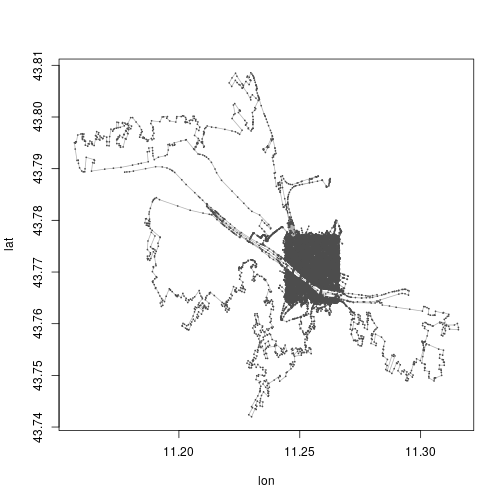Note that what we get a plot of, is, among other things, of all lines that are partly in the box. If a line extends beyond the box, we get it as well.

Looking at the data:

summary(florence$ways)  ## osmar$ways object
## 6707 ways, 9689 tags, 59052 refs
##
## ..$attrs data.frame: ## id, visible, timestamp, version, changeset, user, uid ## ..$tags data.frame:
##     id, k, v
## ..$refs data.frame: ## id, ref ## ## Key-Value contingency table: ## Key Value Freq ## 1 building yes 4157 ## 2 oneway yes 456 ## 3 highway pedestrian 335 ## 4 highway residential 317 ## 5 bicycle yes 316 ## 6 psv yes 122 ## 7 highway unclassified 108 ## 8 highway footway 101 ## 9 barrier wall 98 ## 10 surface paving_stones 87  I would like to plot the roads and buildings. For some reason there are a lot of highways, of a kind I would probably not call highways. Anyway, lets make a list of tags. tags() finds the elements that have a key in the tag_list, way finds the lines that are represented by these elements, and find, finds the ID of the objects in “florence” matching this. find_down() finds all the elements related to these id’s. And finally we take the subset of the large florence data-set, which have id’s matching the id’s we have in from before. tag_list <- c("highway", "bicycle", "oneway", "building") dat <- find(florence, way(tags(k %in% tag_list))) dat <- find_down(florence, way(dat)) dat <- subset(florence, ids = dat)  Now, in a couple of lines, I’m gonna tidy the data. That removes the information of the type of line. As I would like to be able to color highways differently from buildings, I need to keep the information. Saving the key-part of the tags, and the id: types <- data.frame(dat$ways$tags$k, dat$ways$tags$id) names(types) <- c("type", "id")  This gives me all the key-parts of all the tags. And I’m only interested in a subset of them: types <- types %>% filter(type %in% tag_list) types$id <- as.character(types$id)  Next as_sp() converts the osmar object to a spatial object (just taking the lines): dat <- as_sp(dat, "lines")  tidy (from the library broom), converts it to a tidy tibble dat <- tidy(dat)  That tibble is missing the types – those are added. new_df <- left_join(dat, types, by="id")  And now we can plot: new_df %>% ggplot(aes(x=long, y=lat, group=group)) + geom_path(aes(color=type)) + scale_color_brewer() + xlim(left,right) + ylim(bottom,top) + theme_void() + theme(legend.position="none")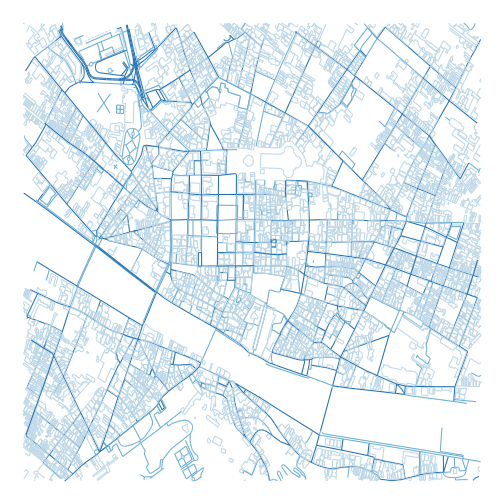Nice. Whats next? Someting like what is on this page: https://github.com/ropensci/osmplotr # Waffle charts A rather popular chart type. Not really my favorite, but I can see how it makes things easier to understand for people who are not used to read and understand charts. The reason for my less than favourable view on waffle charts are probably linked to its overuse in meaningless infographics. A waffle chart is a grid with squares/cells/icons/whatever, where each cell represents a number of something. Lets make an example: library(ggplot2) library(waffle)  vec <- c(Category 1 (10)= 10 , Category 2 (20)= 20, Category 3 (25)= 24, Category 4 (16) = 16) waffle(vec/2, rows=3, size=0.1, colors=c("#c7d4b6", "#a3aabd", "#a0d0de", "#97b5cf"), title="Four different categories of something", xlab="1 square = 2 somethings")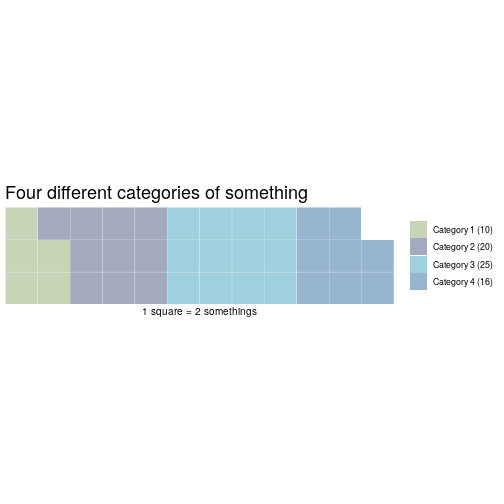One annoyance: waffle wants you to spell colours wrong. waffle takes a named vector of values, rows sets the number of rows of blocks. Default is 10. One standard way, is to show a 10×10 grid, where each cell represents 1% of the total: waffle(vec/sum(vec)*100)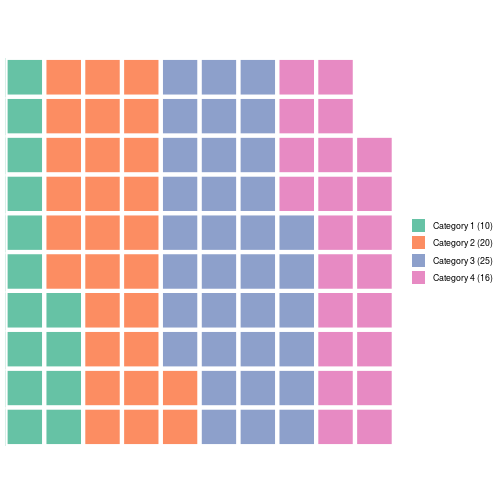Bloody annoying – waffle rounds the values of the vector, leading to only 98 squares. So you have to manipulate your vector to get to 100. Well, actually it is probably a minor annoyance. What if you want something else than coloured squares? The arguments “use_glyph” and “glyph_size” makes that possible. First, we’ll need the library extrafont library(extrafont)  We’ll also need to have the “awesomefonts” installed. It can be downloaded from: http://maxcdn.bootstrapcdn.com/font-awesome/4.3.0/fonts/fontawesome-webfont.ttf This should be easier if you are on a desktop machine. As I’m running this through my own installation of RStudio on a remote server, it was a bit more difficult. I needed to place the “fontawesome.ttf” file in the “/usr/share/fonts/truetype/fontawesome” directory. Then, running R as superuser on the commandline, I imported the extrafont library, and then ran “font_import()”. But then it worked! There is now a long list of 593 different icons, that can be used. If you want a list, just run fa_list(). And now, we can make a waffle chart with the glyph of our choice. waffle(vec/2, rows=4, use_glyph = "wifi")  ## Warning: Removed 1 rows containing missing values (geom_text).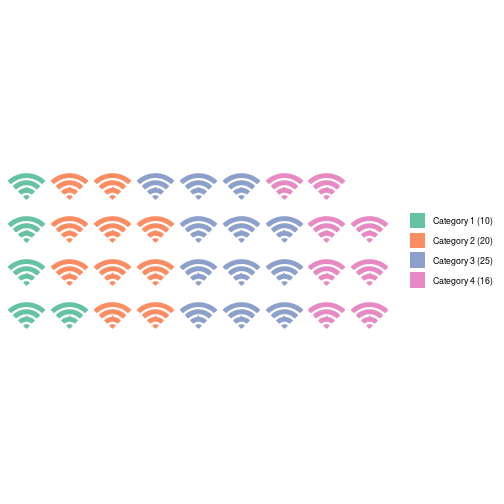We can change the colours: library(RColorBrewer) waffle(vec/2, rows=4, use_glyph = "wifi", colors=brewer.pal(6,"Set1"))  ## Warning: Removed 1 rows containing missing values (geom_text).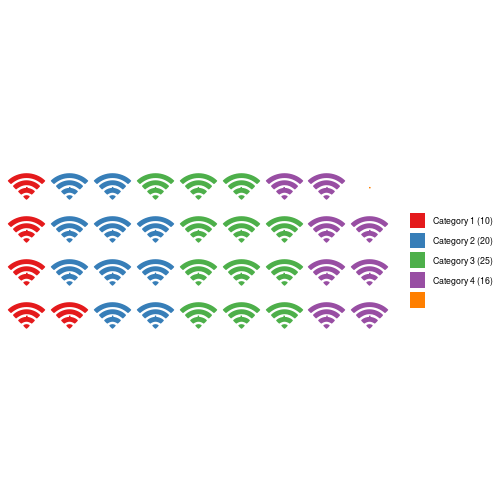Adjust the size waffle(vec/2, rows=4, use_glyph = "wifi", colors=brewer.pal(6,"Set1"), glyph_size=5)  ## Font Awesome by Dave Gandy - http://fontawesome.io  ## Warning: Removed 1 rows containing missing values (geom_text).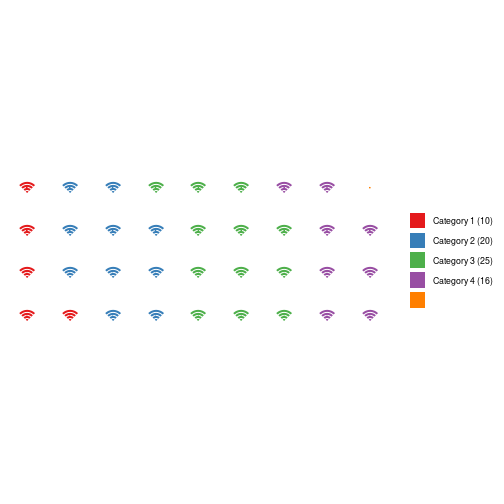But that does not look very good. waffle is based on ggplot, so we have access to the full range of functionality. But not all of them are going to look good in this context: waffle(vec/2, rows=4, use_glyph = "wifi", colors=brewer.pal(4,"Set1")) + geom_label(label="42", size = 3)  ## Warning: Removed 1 rows containing missing values (geom_text).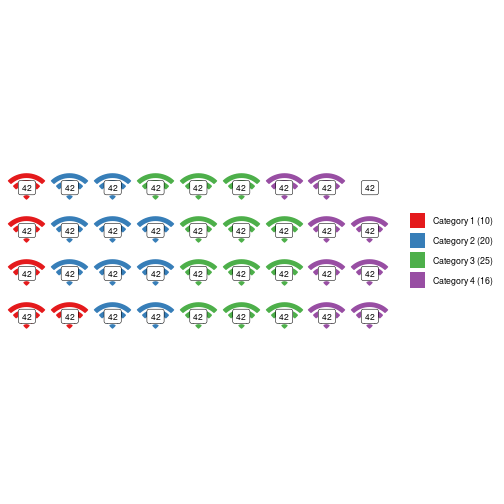If we install a font that supports it, we even get access to the large number of UTF-8 glyphs. Here is a favorite of mine: waffle(vec/2, rows=4, colors=brewer.pal(4,"Set1")) + geom_label(label=sprintf("\U1F427"), size = 8)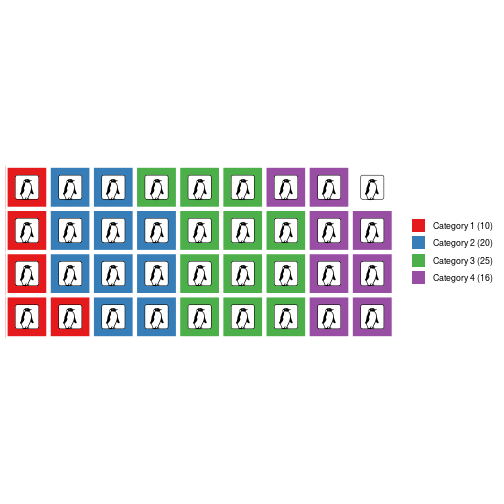Which of course requires you to have a font on your computer that supports penguins. Here is one: http://users.teilar.gr/~g1951d/Symbola.zip Posted in R # Get the font colour from a cell in Excel People do weird and wonderful things in Excel. Other people then have to pull out the data from those spreadsheets. “Other people” tend to spend a lot of time crying into their coffee. At the moment, I am trying to pull out data of a spreadsheet, where “something” can have a value of 1, 2 or 3. That is of course marked by an “x” in a cell. I need to convert that x to a number. That is rather simple. What is not so simple, is that there can be two x’es. One, in black, to denote the current state of affairs. And a second x, in red, to denote what a future, state is wanted to be. So – I need a way to get the color of an x. VBA can do that: Function GetColour(ByVal Target As Range) As Single Application.Volatile GetColour = Target.Font.Color End Function And if I need a logical test: Function IsBlack(ByVal Target As Range) As Boolean Application.Volatile If Target.Font.Color = 0 Then IsBlack = True Else IsBlack = False End If End Function # My biggest weakness? This probably sounds like humble bragging. But I have recently – again – reailized that my biggest weakness is that I take responsibility. Hey! How is that a weakness? Well… It becomes a weakness when you continually take responsibility for stuff that is really not your responsibilty. To the extent that you get stress, hypertension and ulcers. And to the extent that it impacts negatively on the things that actually are your responsibility. And I have just done it again. The ad for a meeting in the local party is not very readable. That is not my responsibility. It belongs to the chairman. Not me. I should simply notify him that it is not very readable. And trust that he will do something about it. Instead I am thinking about remaking it myself. It would not be very difficult. But I do get stressed. If I have to redesign the ad, I wont have time to cook dinner tonight. And clean the house. This is something that I really have to get better at handling. Otherwise I’ll be a very responsible person, doing great things for people and organizations around me. While burning out very fast. # Crimes against graphs Crime is a bad thing. No doubt about it. And one of the main topics in todays debate climate is – “those ‘orrible immigrants are very criminal. Look at these numbers, they prove it!”. Usually written with caps-lock engaged. Well. Maybe they are, and maybe they do. But if you want to use statistics to prove it – pretty please, do not obfuscate the numbers. This is an example. A blog post from one of the more notable danish newspapers. In the US it would be regarded as communist, in the rest of the world we would think of it as relatively conservative. https://kulturkamp.blogs.berlingske.dk/2018/08/17/anmeldte-voldtaegter-og-voldsforbrydelser-er-paa-det-hoejeste-nogensinde/ The claim is, that the number of reported rapes and other violent crimes in Denmark, are the highest ever. That is because of the increasing numbers of immigrants in Denmark, especially muslims. Use Google translate if you want the details. Again, that claim might be true. But the graphs in the post, that supposedly documents the claim, are misleading. To say the least. First – the numbers come from the Danish Statistical Bureau. They have a disclaimer, telling us that changes to the danish penal code, means that a number of sexual offenses have been reclassified as violent crimes since 2013. If the number of violent crimes suddenly includes crimes that did not use to be classified as violent crimes, that number will increase. Not much of a surprise. Yes, the post asks why the numbers are still increasing after that reclassification. One should expect them to level off. And again the post may have a valid point. I don’t know. But what I do know, is that the graphs are misleading. Heres why. The y-axis has been cut of. Lets recreate the graphs, and take a look. There are two graphs. The first shows the number of reported cases of rape from 1995 until today. The second shows the total number of reported cases of violent crimes in the same period. Both sets of data comes from http://www.statistikbanken.dk/. We’re going to need some libraries: library(ggplot2) library(gridExtra)  Lets begin by pulling the data. There might be better ways, but I’ve simply downloaded the data. Two files: violence <- read.csv("tab1.csv", sep=";", skip=3, header=F) rape <- read.csv("tab2.csv", sep=";", skip=3, header=F) violence <- violence[1:(nrow(violence)-7),] rape <- rape[1:(nrow(rape)-7),]  The last seven lines are the notes about changes in which cases are counted in this statistics. I think that is a pretty important point, but they are difficult to plot. The graph for rape, as presented in the post, and with a more sensible y-axis: post <- ggplot(rape, aes(x=V1, y=V2)) + geom_line(group=1) + scale_x_discrete(breaks = rape$V1[seq(1, length(rape$V1), by = 20)]) + theme_classic() nice <- post + ylim(0,max(rape$V2))
grid.arrange(post, nice, ncol=2)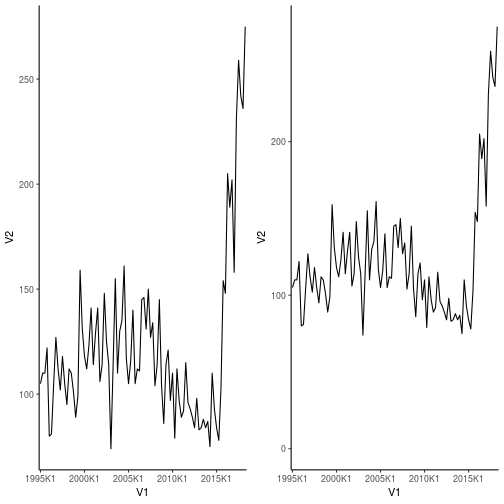And the one for violent crimes in general, again with the original on the left, and the better on the right:

post <- ggplot(violence, aes(x=V1, y=V2)) +
geom_line(group=1) +
scale_x_discrete(breaks = violence$V1[seq(1, length(violence$V1), by = 20)]) +
theme_classic()

nice <- post + ylim(0,max(violence$V2)) grid.arrange(post, nice, ncol=2)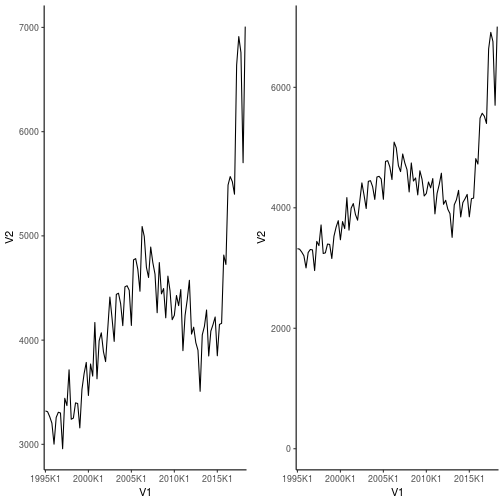So, still, some pretty scary increases. And the change in what is counted should give an increase. But that increase should level off, which it does not. Clearly something is not as it should be. But lets be honest, the graphs on the right are not quite as scary as the ones on the left. Also – that change in what is counted as sexual assaults – it can explain the initial increase, but then it should level off. That is a fair point. However, there were other things that changed in the period. #metoo for example. I think it would be reasonable to expect that a lot of cases that used to be brushed of as not very important, are now finally being reported. The numbers might actually have leveled off without #metoo. Anyway, my point is, that if you want to use graphs to support your claims, do NOT cut off the y-axis to make them look more convincing. Posted in R # Migrants visualized First of all, this is in no way a statement on the immigration crisis in Europe. I do have opinions. But it is more a reaction or reflection on three maps I saw on this page. Danish televison channel TV2 is illustrating the number of refugees or perhaps rather immigrants received in EU-memberstates in the period 2015 to 2017. This is the map showing the number of immigrants to EU in 2015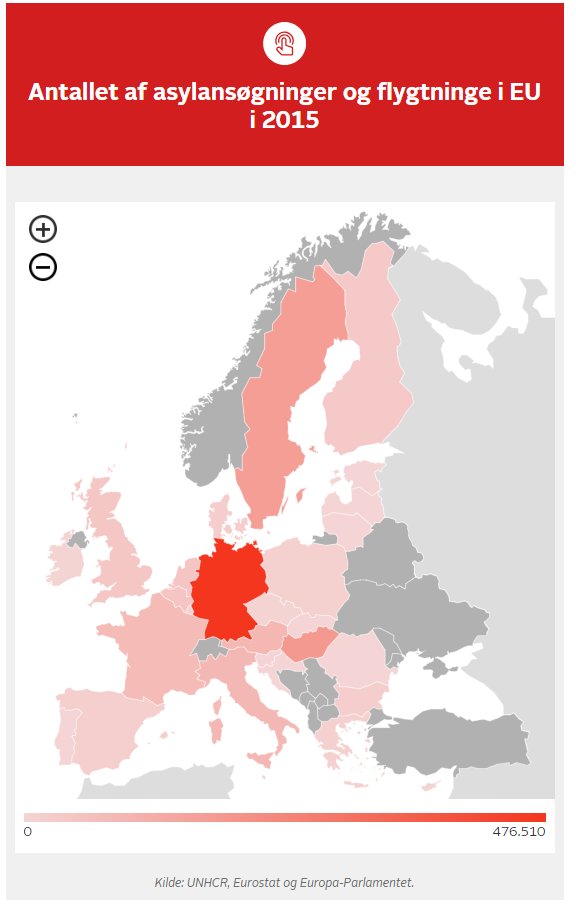Note Germany. Germany welcomed the absolutely highest number of immigrants. What piqued my interest though, is that this might be a good illustration of the numbers, it is not really the relevant comparisons. Yes, Germany welcomed more refugees than Denmark did. But Germany is a rather larger country than Denmark. For a given value of “fair”, it is only fair that Germany takes more refugees than smaller countries. A more relevant comparison might be the number of refugees compared to population. Or area. Sweden saw (at that time) no problems with welcoming a huge number of migrants, because, as they said, there are a lot of un-populated space in Sweden, plenty of room for everyone! Or perhaps GDP is a better way. Richer countries should shoulder a larger part of the challenge than poorer countries. I’m not concerned here with what is fair. What concerns me is that the graphic is misleading. Lets make an attempt at fixing that. Or at least present a slightly different perspective on the data. I’ll try to illustrate the number of migrants as a proportion of population in the different countries. The data is “stolen” directly from the news-channel. They have it from UNHCR, Eurostat and the European Parlament. The first step will be to get the data. url <- "http://nyheder.tv2.dk/udland/2018-06-28-se-kortet-saa-mange-asylansoegere-har-de-forskellige-eu-lande-taget" data <- readLines(url)  By inspection, I can see that the relevant data is in these three lines: dat.2015 <- data dat.2016 <- data dat.2017 <- data  There is a small problem. Strange danish characters are encoded. Lets fix that: library(stringr) data <- str_replace_all(data,"\\\\u00d8", "Ø") data <- str_replace_all(data,"\\\\u00e6", "æ") data <- str_replace_all(data,"\\\\u00f8", "ø")  And load it into the separate variables again: dat.2015 <- data dat.2016 <- data dat.2017 <- data  Next, I need to get the names of the countries, and the number of migrants received in each country. The data I’m after looks like this: \“Bulgarien\”:{\“valueheat\”:20365,\“valuecolored\”:\“none\”,\“description\”:\“\”}, And the regular expression picking that out of the data ought to be: ‘\“(\p{L}+)\”:{\“valueheat\”:(\d+|\“\”),’ For some reason that is not working. I probably should try to figure that out, but I’m on vacation, and would rather drink cold white wine that dig too deep into the weirdness that is regular expressions in R. Instead I’m going to use this simpler pattern: pattern <- '(\")(\\w+)(.*?)(\\d+|\\\"\\\")'  And then fix problems later. Extracting the data: dat.2015 <- unlist(str_extract_all(dat.2015, pattern)) dat.2016 <- unlist(str_extract_all(dat.2016, pattern)) dat.2017 <- unlist(str_extract_all(dat.2017, pattern))  Inspecting the data, reveals that the interesting parts are lines 23 to 111. dat.2015 <- dat.2015[23:111] dat.2016 <- dat.2016[23:111] dat.2017 <- dat.2017[23:111]  An example of two lines: dat.2015[11:12]  ##  "\"Danmark\":{\"valueheat\":20935" ##  "\"valuecolored\":\"none\",\"description\":\"\""  First, lets get rid of the second line. There are one of those for each country. Secondly, I’ll remove the first to characters in the first line. \“ to be precise. And thirdly, I’ll split that line on \”:{\“valueheat\”: Using the nice pipes makes that easy: library(purrr) dat.2015 <- dat.2015 %>% discard(str_detect, "valuecolored") %>% substring(2) %>% strsplit(split="\":{\"valueheat\":",fixed=TRUE)  That should give me a list where each element is a vector with two elements: dat.2015[]  ##  "Finland" "32345"  Neat! Lets do that with the other years: dat.2016 <- dat.2016 %>% discard(str_detect, "valuecolored") %>% substring(2) %>% strsplit(split="\":{\"valueheat\":",fixed=TRUE) dat.2017 <- dat.2017 %>% discard(str_detect, "valuecolored") %>% substring(2) %>% strsplit(split="\":{\"valueheat\":",fixed=TRUE)  Now, lets get these data into some dataframes. First I’m unlisting the data, then I pour it into a matrix to get the right shape. And then I’m converting the matrices to dataframes: dat.2017 <- data.frame(matrix(unlist(dat.2017), ncol=2, byrow=T), stringsAsFactors = F) dat.2016 <- data.frame(matrix(unlist(dat.2016), ncol=2, byrow=T), stringsAsFactors = F) dat.2015 <- data.frame(matrix(unlist(dat.2015), ncol=2, byrow=T), stringsAsFactors = F)  There is a slight problem. Albania was the first country in the list. And the structure of the raw data was a bit different. dat.2015[1,1]  ##  "regions\":{\"Albanien"  Lets fix that: dat.2015[1,1] <- "Albanien" dat.2016[1,1] <- "Albanien" dat.2017[1,1] <- "Albanien"  And let me just change the column names: colnames(dat.2015) <- c("Land", "2015") colnames(dat.2016) <- c("Land", "2016") colnames(dat.2017) <- c("Land", "2017")  “Land” is danish for “country”. I’m going to need just one dataframe. I get that by joining the three dataframes: library(dplyr) total <- left_join(dat.2015, dat.2016, by="Land") total <- left_join(total, dat.2017, by="Land")  The numbers are saved as characters. Converting them to numeric: total$2015 <- as.numeric(total$2015)  ## Warning: NAs introduced by coercion  total$2016 <- as.numeric(total$2016)  ## Warning: NAs introduced by coercion  total$2017 <- as.numeric(total$2017)  ## Warning: NAs introduced by coercion  That introduced some NAs. Countries where there are no data. Inspecting the data, I can see that there are data for all three years for some countries. For other countries, there are no data at all. The function complete.cases() will return true for a row without NAs. Using that to get rid of countries where we don’t have complete data: total <- total[complete.cases(total),]  Next is getting some figures for the populations. The relevant page on Wikipedia is: url <- 'https://da.wikipedia.org/wiki/Verdens_landes_befolkningsst%C3%B8rrelser'  Getting that: library(XML) library(httr) r <- GET(url) doc <- readHTMLTable( doc=content(r, "text")) tabellen <- doc$'NULL'

colnames(tabellen) <-  apply(tabellen[1,],2,as.character)


I’m only interested in the country name, and the population:

tabellen <- tabellen %>%
select(Land (eller territorium), Population)


Renaming the colums:

colnames(tabellen) <- c("Land", "Population")
tabellen$Population <- as.character(tabellen$Population)
tabellen$Population <- as.numeric(str_remove_all(tabellen$Population, fixed(".")))

## Warning: NAs introduced by coercion


And while I’m at it, the second line gets rid of the factors, and the third removes the thousand separators (“.”)

Now I can join the dataframe containing population figures, with the dataframe containing countries and number of migrants:

total <- left_join(total, tabellen, by="Land")

## Warning: Column Land joining character vector and factor, coercing into
## character vector


There are three smaller problems. Cyprys, France and Ireland. The problem is that the country name I get from Wikipedia contains a note. I might be able to get rid of that by code. I’m going to do it manually.

total[10,5] <- 4635400
total[7,5] <- 67286000
total[3,5] <- 847000


Now I have a nice dataframe with the name of the countries (in danish), the numbe of migrants received in 2015, 2016 and 2017, and the population in 2018.

Now it is time to look at some maps.

library(ggplot2)
library(rworldmap)

## ### Welcome to rworldmap ###

## For a short introduction type :   vignette('rworldmap')


I am going to match the countries in my dataframe, with the countries I get from the map data. That requires that I have the english names for the countries in my dataframe.

This is the translation:

enland <- c("Belgium", "Bulgaria", "Cyprus", "Denmark", "Estonia", "Finland", "France", "Greece", "Netherlands", "Ireland",
"Italy", "Croatia", "Latvia", "Lithuania", "Luxembourg", "Malta", "Poland", "Portugal", "Romania", "Slovakia",
"Slovenia", "Spain", "United Kingdom", "Sweden", "Czech Rep.", "Germany", "Hungary", "Austria"  )


Getting that into the data frame:

total$enland <- enland  Next, lets get the map: worldmap <- getMap()  That retrieves data for the entire world. I’m only interested in EU: EU <- worldmap[which(worldmap$NAME %in% enland),]
EU <- map_data(EU)

##
## Attaching package: 'maps'

## The following object is masked from 'package:purrr':
##
##     map


The first line extracts the part of the world map that has names in the list of countries that I have data for.
map_data() converts that into a nice structure that is suitable for entering into ggplot.

Next step is calculating the number of migrants received in each country as a proportion of that countrys population:

total <- total %>%
mutate(2015 = 2015/Population*100, 2016 = 2016/Population*100, 2017=2017/Population*100)


I’m mutating the columns 2015-2017 by dividing by population. And multiplying by 100 to get percentages.

The almost final step, is to join my migrant-proportions with the map data:

total <- left_join(total,EU, by=c("enland"="region") )


The map data does not call the countries for countries. Rather their names are saved in the variable “region”.

And now the final step. I’m going to need the data on tidy form. So I’m loading tidyr.

Then I pass the data frame to select(), where I pick out the variables I need. Long(itude), lat(itude), 2015, 2016 and 2017, and the name of the country.
That is passed to gather(), where I make a new row for each year, with the proportions in the new variabels year and prop.

All that is passed to ggplot, and a layer where the polygons describing the countries are plotted. They are with a colour matching the proportions. And grouped by “group”. This is important. Grouping by country name gives weird results. I’ll get back to that. color=“white” plots the lines in the polygons in white.

Finally, I facet the data on year.

library(tidyr)
total %>%
select(long,lat,2015,2016,2017, group) %>%
gather(year, prop,  2015:2017)%>%
ggplot() +
geom_polygon(aes(long, lat, fill = prop, group = group), color = "white") +
theme_void() +
facet_wrap(~year, ncol=2)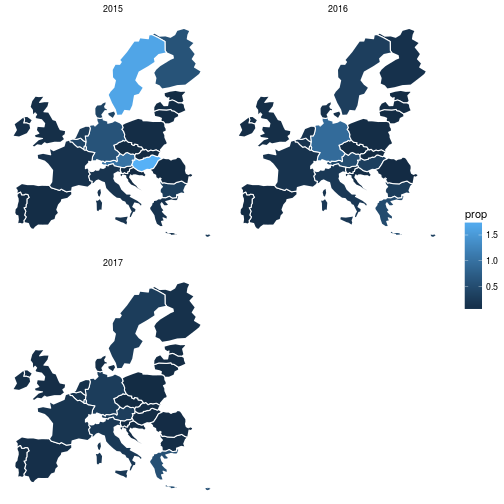Thats it!
And now the picture is slightly different. What is interesting is that Germany still takes a higher proportion of the migrants than other countries. But in 2015, they didn’t. That was the year when the german chancellor Angela Merkel said the famous words “Wir schaffen das”, We’ll manage. But also the year when Hungary and sweden welcomed migrants in numbers equalling 1.79% and 1.65% of their population respectively. You can compare that with the fact that Germany the same year received migrants equalling 0.58% of their population.

A cynic might claim that it is no surprise that Sweden and Hungary closed their borders late in 2015.

Any way, that is a different subject. I just think that these three maps are slightly more informative than what TV2 provided.

Also, I promised to get back to the group thingy.

Making the same plot, but grouping on country names:

total %>%
select(long,lat,2015,2016,2017, group, enland) %>%
gather(year, prop,  2015:2017)%>%
ggplot() +
geom_polygon(aes(long, lat, fill = prop, group = enland), color = "white") +
theme_void()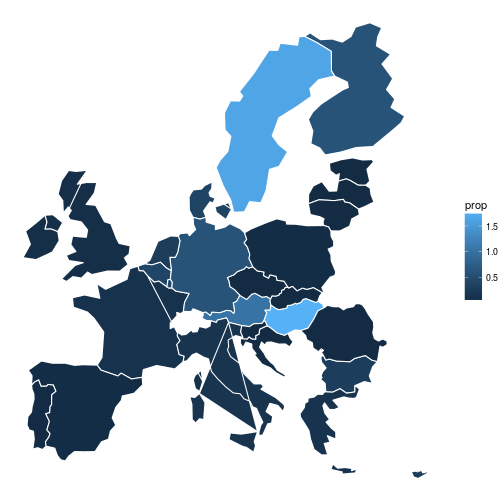What happens is that the polygons describing Italy are grouped in a way that connects the parts describing sicily to the northern part of Italy. That looks weird. The same happens with Sardinia.

Finally. I have not been very consistent in my use of words. I have used “received” and “welcomed” interchangeably. Hungary and Denmark has not been very welcoming. But we are talking about real humans here, and welcoming simply sounds nicer than received. Complicating the situation was the fact that a lot of the arrivals were not actually what we would normally call refugees. At least not refugees from war. So I have also not been consistent in the use of “migrant” vs “refugee”. That is not really my point. The point is that we should always think about how these kinds of numbers are presented.

Posted in R